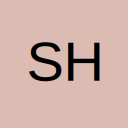# Choosing the array name based on a value in a cell

• Hi,

I'm trying to use a sumproduct formula but I would like the name of the array to be chosen by a value in a cell, I don't want to type it in the formula because it changes often.

Attached is a sample of the file that I'm working on. I would like to name the arrays by the date that they pertain to, i.e. 04/11/2012 and then I would like the formula to choose the array based on the value in column B. Currently my arrays are named, "Nov12_wk1", "Nov12_wk2" etc but I would like to name then according to the date that they pertain to.

Thanks,

Sherie

## Files

• Re: Choosing the array name based on a value in a cell

you can use indirect
=SUMPRODUCT((INDEX(INDIRECT(K4),1,0)=D\$2)*(INDEX(INDIRECT(K4),0,1)=\$C3),INDIRECT(K4))
I have put Nov12_wk1 in cell K4
and then changed your formula to use K4 as the name range

i'm not sure if you can name an array based on a dateformat - you need a Letter/_ and cannot use a default excel name to use

so you may need to think about how you can change the date to use as a named range

That's exactly what I was looking for, thanks so much